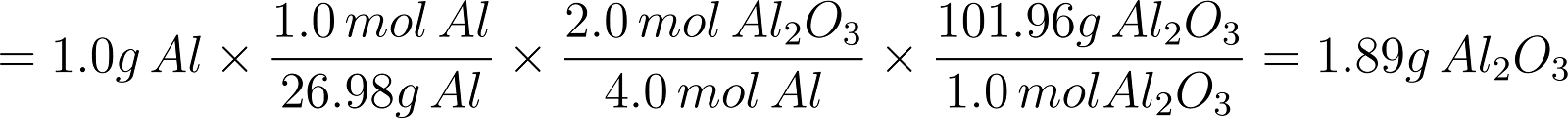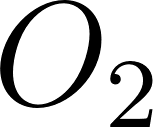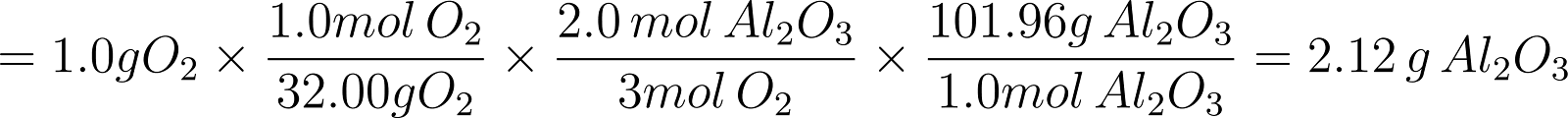×
Get Full Access to Introductory Chemistry - 5 Edition - Chapter 8 - Problem 56p
Get Full Access to Introductory Chemistry - 5 Edition - Chapter 8 - Problem 56p

×

# For the reaction, find the limiting reactant for each of the initial quantities ofISBN: 9780321910295 34

## Solution for problem 56P Chapter 8

Introductory Chemistry | 5th Edition

• Textbook Solutions
• 2901 Step-by-step solutions solved by professors and subject experts
• Get 24/7 help from StudySoup virtual teaching assistantsIntroductory Chemistry | 5th Edition

4 5 1 332 Reviews
12
0
Problem 56P

PROBLEM 56P

For the reaction, find the limiting reactant for each of the initial quantities of reactants.

4 Al(s) + 3 O2(g) → 2 Al2O3(s)

(a) 1.0 g Al; 1.0 g O2

(b) 2.2 g Al; 1.8 g O2

(c) 0.353 g Al; 0.482 g O2

Step-by-Step Solution:

PROBLEM 56P

For the reaction, find the limiting reactant for each of the initial quantities of reactants.(a) 1.0 g Al; 1.0 g O2

(b) 2.2 g Al; 1.8 g O2

(c) 0.353 g Al; 0.482 g O2

Step by Step Solution

Step 1 of 3

a)

The given chemical reaction is as follows.Let’s calculate the amount ofwas produced if “Al” is the limiting reactant.Hence, 1.89 g ofwas produced if “Al” is the limiting reactant.

Let’s calculate the amount ofwas produced ifis the limiting reactant.Hence, 2.12 g ofwas produced ifis the limiting reactant.

From the above calculations “Al” produces the least amount of product.

Therefore, “Al” is the limiting reactant.

___________________________________________________________________________

Step 2 of 3

Step 3 of 3

##### ISBN: 9780321910295

Introductory Chemistry was written by and is associated to the ISBN: 9780321910295. This full solution covers the following key subjects: Find, initial, limiting, quantities, reactant. This expansive textbook survival guide covers 19 chapters, and 2046 solutions. The answer to “For the reaction, find the limiting reactant for each of the initial quantities of reactants.4 Al(s) + 3 O2(g) ? 2 Al2O3(s)(a) 1.0 g Al; 1.0 g O2(b) 2.2 g Al; 1.8 g O2(c) 0.353 g Al; 0.482 g O2” is broken down into a number of easy to follow steps, and 40 words. The full step-by-step solution to problem: 56P from chapter: 8 was answered by , our top Chemistry solution expert on 05/06/17, 06:45PM. This textbook survival guide was created for the textbook: Introductory Chemistry, edition: 5. Since the solution to 56P from 8 chapter was answered, more than 418 students have viewed the full step-by-step answer.

Unlock Textbook Solution